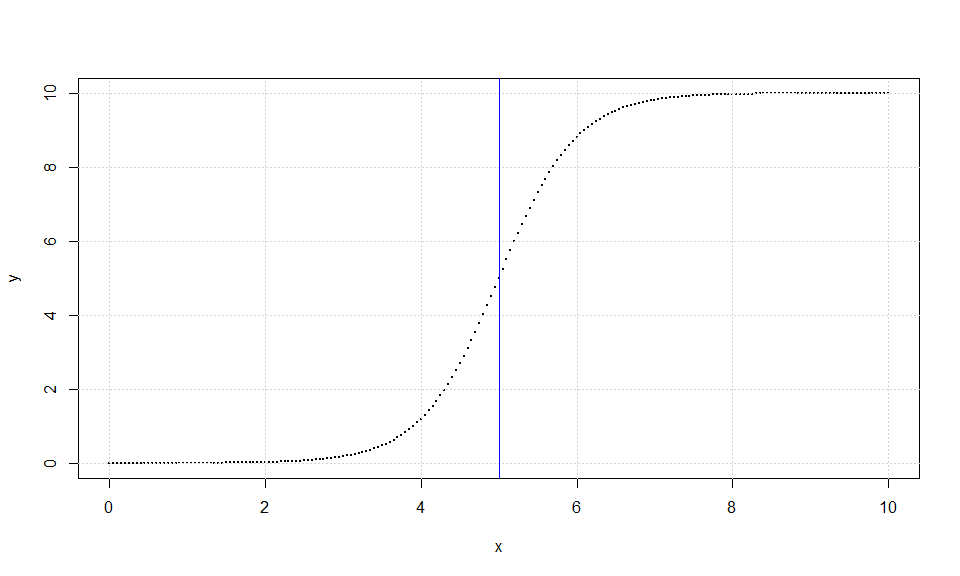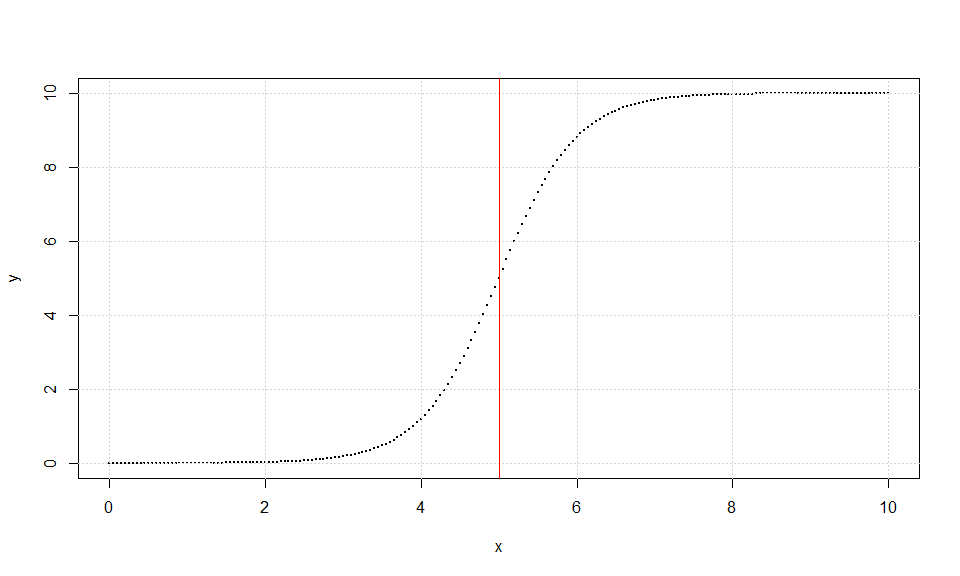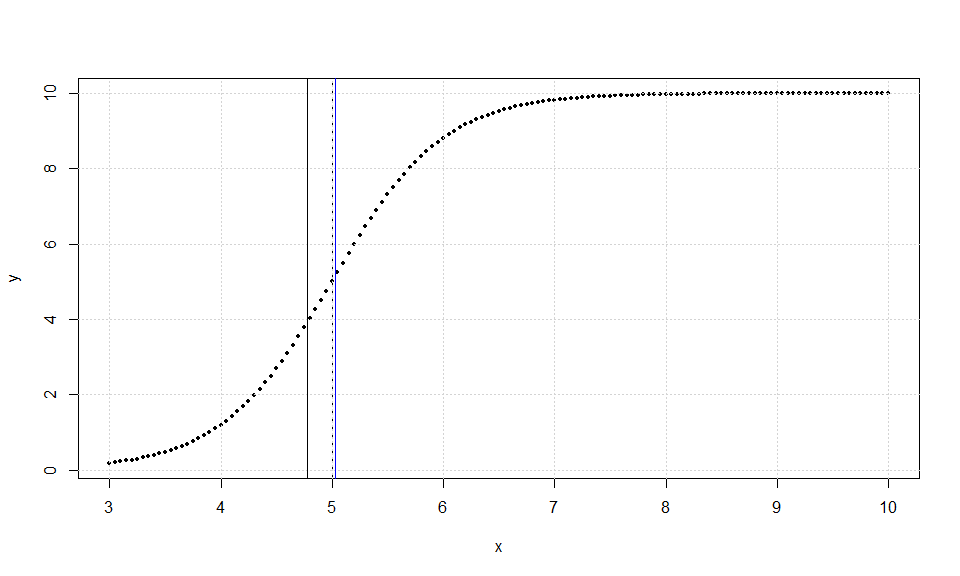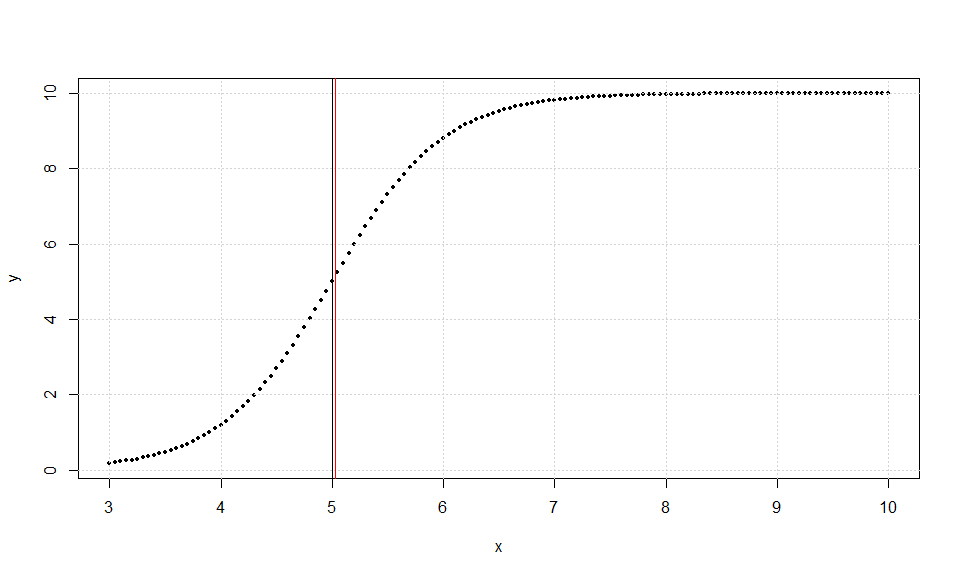# Introduction to inflection package

## Inflection Point

When a planar curve is first convex and then concave or vice versa, then there exists an inflection point (ip). We could find that if all our points had no error, but that’s an exception to reality. Since the divided differences of second order are extremely sensitive to the error, it is meaningless to use them as an approximation in order to search for inflection point. For that task Extremum Surface Estimator (ESE), Extremeum Distance Estimator (EDE) and their iterative versions Bisection ESE (BESE), Bisection EDE (BEDE) have been created, see  &  for definitions and properties.

Lets create a not noisy data set from function $$f(x)=5+5\,tanh(x-5)$$ with known inflection point $$(ip=5)$$, equal x-distances and total symmetry, see . Actually it is the data set “TABLE_2_1” of package.

### Now we compute the results of all available methods for non noisy data sets

#### ESE & BESE

f=function(x){5+5*tanh(x-5)}
x=seq(0,10,by=0.05)
y=f(x)
plot(x,y,cex=0.3,pch=19)
grid()
bb=ese(x,y,0);bb
##     j1  j2 chi
## ESE 77 125   5
pese=bb[,3];pese
##  5
abline(v=pese)
cc=bese(x,y,0)
cc$iplast ##  5 abline(v=cc$iplast,col='blue')knitr::kable(cc$iters, caption = 'BESE') BESE n a b ESE 201 0.00 10.00 5 49 4.45 5.55 5 23 4.75 5.25 5 11 4.90 5.10 5 5 4.95 5.05 5 #### EDE & BEDE x=seq(0,10,by=0.05) y=f(x) plot(x,y,cex=0.3,pch=19) grid() dd=ede(x,y,0);dd ## j1 j2 chi ## EDE 72 130 5 pede=dd[,3];pede ##  5 abline(v=pede) ee=bede(x,y,0) ee$iplast
##  5
abline(v=cc$iplast,col='red')knitr::kable(ee$iters, caption = 'BEDE')
BEDE
n a b EDE
201 0.00 10.00 5
59 4.30 5.70 5
29 4.60 5.40 5
17 4.75 5.25 5
11 4.85 5.15 5
7 4.90 5.10 5
5 4.95 5.05 5

So all methods gave the theoretical expected value. Lets create a data set with data right asymmetry, see  and run again:

x=seq(3,10,by=0.05)
y=f(x)
plot(x,y,cex=0.5,pch=19)
abline(v=5,lty=2)
grid()
bb=ese(x,y,0);bb
##     j1 j2   chi
## ESE 17 56 4.775
pese=bb[,3];pese
##  4.775
abline(v=pese)
cc=bese(x,y,0)
cc$iplast ##  5.025 abline(v=cc$iplast,col='blue')knitr::kable(cc$iters, caption = 'BESE') BESE n a b ESE 141 3.00 10.00 4.775 40 4.65 5.55 5.100 19 4.75 5.15 4.950 9 4.95 5.10 5.025 x=seq(3,10,by=0.05) y=f(x) plot(x,y,cex=0.5,pch=19) abline(v=5,lty=2) grid() dd=ede(x,y,0);dd ## j1 j2 chi ## EDE 16 66 5 pede=dd[,3];pede ##  5 abline(v=pede) ee=bede(x,y,0) ee$iplast
##  5
abline(v=cc$iplast,col='red')knitr::kable(ee$iters, caption = 'BEDE')
BEDE
n a b EDE
141 3.00 10.00 5
51 4.35 5.65 5
27 4.65 5.35 5
15 4.80 5.20 5
9 4.90 5.10 5
5 4.95 5.05 5